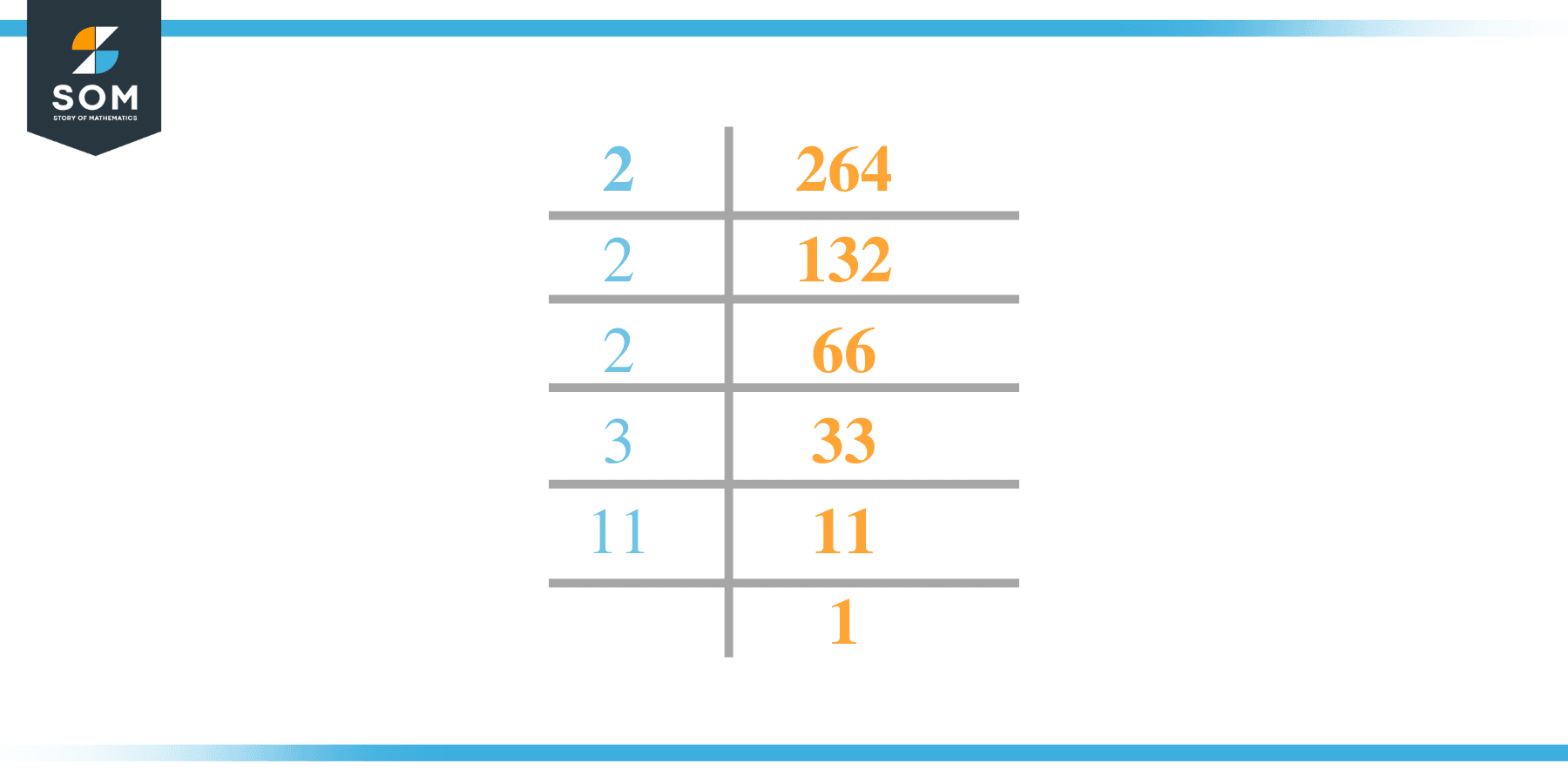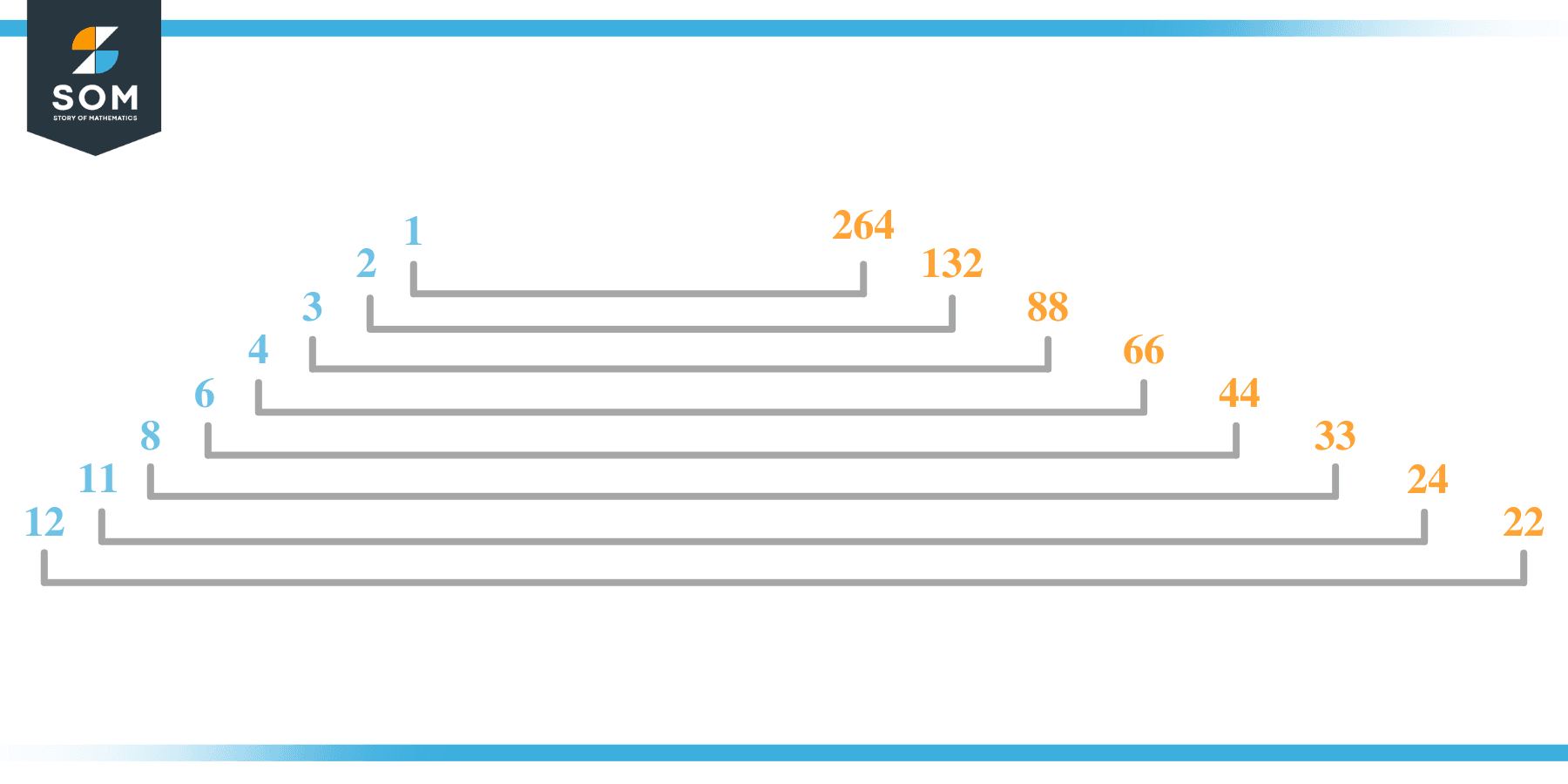# Factors of 264: Prime Factorization, Methods, and Examples

The factors of 264 are numbers that, when divided by 264, leave zero as the remainder. This means the numbers that ultimately divide the given number are named as their factors.The given number’s factors can be positive and negative, provided that the given number is achieved upon multiplication of two-factor integers.

### Factors of 264

Here are the factors of number 264.

Factors of 264: 1, 2, 3, 4, 6, 8, 11, 12, 22, 24, 33, 44, 66, 88, 132, and 264

### Negative Factors of 264

The negative factors of 264 are similar to their positive aspects, just with a negative sign.

Negative Factors of 264: -1, -2, -3, -4, -6, -8, -11, -12, -22, -24, -33, -44, -66, -88, -132, and -264

### Prime Factorization of 264

The prime factorization of 264 is the way of expressing its prime factors in the product form.

Prime Factorization: 2 x 2 x 2 x 3 x 11

In this article, we will learn about the factors of 264 and how to find them using various techniques such as upside-down division, prime factorization, and factor tree.

## What Are the Factors of 264?

The factors of 264 are 1, 2, 3, 4, 6, 8, 11, 12, 22, 24, 33, 44, 66, 88, 132, and 264. These numbers are the factors as they do not leave any remainder when divided by 264.

The factors of 264 are classified as prime numbers and composite numbers. The prime factors of the number 264 can be determined using the prime factorization technique.

## How To Find the Factors of 264?

You can find the factors of 264 by using the rules of divisibility. The divisibility rule states that any number, when divided by any other natural number, is said to be divisible by the number if the quotient is the whole number and the resulting remainder is zero.

To find the factors of 264, create a list containing the numbers that are exactly divisible by 264 with zero remainders. One important thing to note is that 1 and 264 are the 264’s factors, as every natural number has 1 and the number itself as its factor.

1 is also called the universal factor of every number. The factors of 264 are determined as follows:

$\dfrac{264}{1} = 264$

$\dfrac{264}{2} = 132$

$\dfrac{264}{3} = 88$

$\dfrac{264}{4} = 66$

$\dfrac{264}{6} = 44$

$\dfrac{264}{8} = 33$

$\dfrac{264}{11} = 24$

$\dfrac{264}{12} = 22$

$\dfrac{264}{264} = 1$

Both the divisors and dividends are the factors of  264. Therefore, 1, 2, 3, 4, 6, 8, 11, 12, 22, 24, 33, 44, 66, 88, 132, and 264.

### Total Number of Factors of 264

For 264, there are n positive factors and n negative ones. So in total, there are m factors of 264.

To find the total number of factors of the given number, follow the procedure mentioned below:

1. Find the factorization/prime factorization of the given number.
2. Demonstrate the prime factorization of the number in the exponent form.
3. Add 1 to each of the exponents of the prime factor.
4. Now, multiply the resulting exponents together. This obtained product is equivalent to the total number of factors of the given number.

By following this procedure, the total number of factors of 264 is given as:

Factorization of 264 is 1 x 2$^3$ x 3 x 11.

The exponent of 2 is 3, whereas the exponents of 1, 3, and 11 are 1.

Adding 1 to each and multiplying them together results in 32.

Therefore, the total number of factors of 264 is 32. 16 are positive, and 16 factors are negative.

### Important Notes

Here are some essential points that must be considered while finding the factors of any given number:

• The factor of any given number must be a whole number.
• The number factors cannot be in the form of decimals or fractions.
• Factors can be positive as well as negative.
• Negative factors are the additive inverse of the positive factors of a given number.
• The factor of a number cannot be greater than that number.
• Every even number has 2 as its prime factor, the smallest prime factor.

## Factors of 264 by Prime FactorizationThe number 264 is a composite. Prime factorization is a valuable technique for finding the number’s prime factors and expressing the number as the product of its prime factors.

Before finding the factors of 264 using prime factorization, let us find out what prime factors are. Prime factors are the factors of any given number that are only divisible by 1 and themselves.

To start the prime factorization of 264, start dividing by its most minor prime factor. First, determine that the given number is either even or odd. If it is an even number, then 2 will be the smallest prime factor.

Continue splitting the quotient obtained until 1 is received as the quotient. The prime factorization of 264 can be expressed as:

264 = 2 x 2 x 2 x 3 x 11

## Factors of 264 in PairsThe factor pairs are the duplet of numbers that, when multiplied together, result in the factorized number. Factor pairs can be more than one depending on the total number of factors given.

For 264, the factor pairs can be found as:

1 x 264 = 264

2 x 132 = 264

3 x 88 = 264

4 x 66 = 264

6 x 44 = 264

8 x 33 = 264

11 x 24 = 264

12 x 22 = 264

The possible factor pairs of 264 are given as (1, 264), (2, 132), (3, 88), (4, 66), (6, 44), (8, 33), (11, 24) and (12, 22).

All these numbers in pairs, when multiplied, give 264 as the product.

The negative factor pairs of 264 are given as:

-1 x -264 = 264

-2 x -132 = 264

-3 x -88 = 264

-4 x -66 = 264

-6 x -44 = 264

-8 x -33 = 264

-11 x -24 = 264

-12 x -22 = 264

The possible factor pairs of 264 are given as (-1, -264), (-2, -132), (-3, -88), (-4, -66), (-6, -44), (-8, -33), (-11, -24) and (-12, -22).

It is important to note that in negative factor pairs, the minus sign has been multiplied by the minus sign, due to which the resulting product is the original positive number.

The list of all the factors of 264, including positive as well as negative numbers, is given below.

Factor list of 264: 1, -1, 2, -2, 3, -3, 4, -4, 6, -6, 8, -8, 11, -11, 12, -12, 22, -22, 24, -24, 33, -33, 44, -44, 66, -66, 88, -88, 132, -132, 264 and -264.

## Factors of 264 Solved Examples

To better understand the concept of factors, let’s solve some examples.

### Example 1

How many factors of 264 are there?

### Solution

The total number of Factors of 264 is 16.

Factors of 264 are 1, 2, 3, 4, 6, 8, 11, 12, 22, 24, 33, 44, 66, 88, 132, and 264.

### Example 2

Find the factors of 264 using prime factorization.

### Solution

The prime factorization of 264 is given as:

264 $\div$ 2 = 132

132 $\div$ 2 = 66

66 $\div$ 2 = 33

33 $\div$ 3 = 11

11 $\div$ 11 = 1

So the prime factorization of 264 can be written as:

2 x 2 x 2 x 2 x 3 x 11 = 264